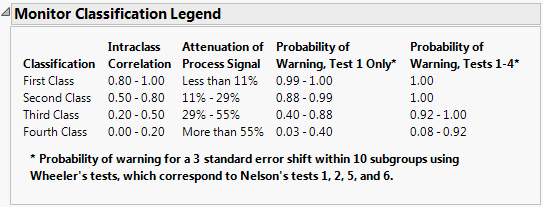Publication date: 07/30/2020

## EMP Results

Note: The statistics in this report are based on ranges in the following instances: if you selected EMP as the MSA Method and Range as the Chart Dispersion Type, and you have a one factor or a two factor, balanced, crossed model. Otherwise, the statistics in this report are based on variances.

The EMP Results report computes several statistics to help you assess and classify your measurement system. Using this report, you can determine the following:

How your process chart is affected.

Which tests to set.

How much the process signal is attenuated.

How much the bias factors are affecting your system and reducing your potential intraclass correlation coefficient.

The EMP Results report contains the following calculations:

Test-Retest Error

Indicates measurement variation or repeatability (also known as within error or pure error).

Degrees of Freedom

Indicates the amount of information used to estimate the within error.

Probable Error

The median error for a single measurement. Indicates the resolution quality of your measurement and helps you decide how many digits to use when recording measurements. See Effective Resolution.

Intraclass Correlation

Indicates the proportion of the total variation that you can attribute to the part. If you have very little measurement variation, this number is closer to 1.

Intraclass Correlation (no bias)

Does not take bias or interaction factors into account when calculating the results.

Intraclass Correlation (with bias)

Takes the bias factors (such as operator, instrument, and so on) into account when calculating the results.

Intraclass Correlation (with bias and interaction)

Takes the bias and interaction factors into account when calculating the results. This calculation appears only if the model is crossed and uses standard deviation instead of range.

Bias Impact

The amount by which the bias factors reduce the Intraclass Correlation.

Bias and Interaction Impact

The amount by which the bias and interaction factors reduce the Intraclass Correlation. This calculation appears only if the model is crossed and uses standard deviation instead of range.

### Classes of Process Monitors

In order to understand the System and Classification parameters, you must first understand the Monitor Classification Legend.

Figure 4.6 Monitor Classification LegendThis legend describes the following classifications: First, Second, Third, and Fourth Class. Each classification indicates the following:

the corresponding Intraclass Correlation values

the amount of process signal attenuation (decrease)

the chance of detecting a 3 standard error shift within 10 subgroups, using Wheeler’s test one or all four tests

Wheeler (2006) identifies four detection tests known as the Western Electric Zone Tests. Within the Shift Detection Profiler, there are eight tests that you can select from. The tests that correspond to the Wheeler tests are the first, second, fifth, and sixth tests.

Tip: To prevent the legend from appearing, deselect Show Monitor Classification Legend in the EMP Measurement Systems Analysis platform preferences.